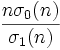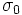# Ore's conjecture

This conjecture states that there are no odd numbers satisfying a certain condition. Typically, there are known to be many even numbers (in some cases, there are known to be infinitely many even numbers) satisfying the condition.
View other such conjectures

## Statement

The conjecture states that the harmonic mean of all the positive divisors of an odd natural number greater thancannot be an integer.

The harmonic mean of all the positive divisors is given by the expression:,

whereis the divisor count function andis the divisor sum function. Natural numbersfor which this ratio is an integer are termed harmonic divisor numbers or Ore numbers, and Ore's conjecture can thus be stated more compactly as: there is no odd Ore number.